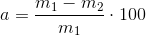## Smelting loss percentage

Percentage rate of the difference in weight between the cold batch and the finished molten metal provided in the furnace, or in other words: the weight loss by oxidation in percent. By way of calculation, the smelting loss percentage is determined by the equation below (Eq. 1):

Eq. 1m1 = weight of cold batch [kg]; m2 = weight of finished molten metal [kg]; a = smelting loss percentage [%]